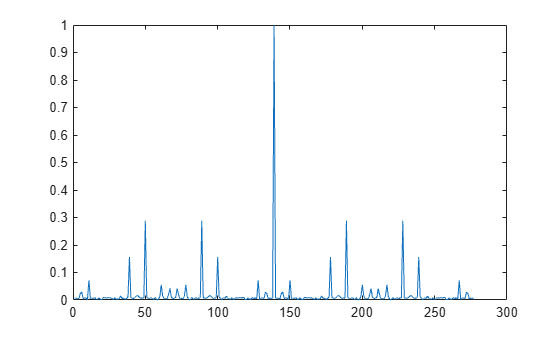## Syntax

``seq = zadoffChuSeq(R,N)``

## Description

example

````seq = zadoffChuSeq(R,N)` generates the `R`th root Zadoff-Chu sequence with length `N`, as defined in 3GPP TS 36.211.The function generates the sequence using the algorithm given byseq(m+1) = exp(-j·π·`R`·m·(m+1)/`N`), for m = 0, ..., `N`-1.The function uses a negative polarity on the argument of the exponent, that is, a clockwise sequence of phases.```

## Examples

collapse all

Generate the 25th root Zadoff-Chu sequence with a length of 139.

Plot the absolute values of the output sequence.

```seq = zadoffChuSeq(25,139); plot(abs(xcorr(seq)./length(seq)))```## Input Arguments

collapse all

Root of the Zadoff-Chu sequence, specified as a positive integer.

Example: `25`

Data Types: `double`

Length of the Zadoff-Chu sequence, specified as an odd positive integer.

Example: `139`

Data Types: `double`

## Output Arguments

collapse all

`R`th root Zadoff-Chu sequence, returned as an `N`-by-1 vector of complex values.

## Compatibility Considerations

expand all

 3GPP TS 36.211. "Evolved Universal Terrestrial Radio Access (E-UTRA); Physical Channels and Modulation." 3rd Generation Partnership Project; Technical Specification Group Radio Access Network..